Courses

# Test: Perimeter And Area - 3

## 20 Questions MCQ Test Mathematics (Maths) Class 7 | Test: Perimeter And Area - 3

Description
This mock test of Test: Perimeter And Area - 3 for Class 7 helps you for every Class 7 entrance exam. This contains 20 Multiple Choice Questions for Class 7 Test: Perimeter And Area - 3 (mcq) to study with solutions a complete question bank. The solved questions answers in this Test: Perimeter And Area - 3 quiz give you a good mix of easy questions and tough questions. Class 7 students definitely take this Test: Perimeter And Area - 3 exercise for a better result in the exam. You can find other Test: Perimeter And Area - 3 extra questions, long questions & short questions for Class 7 on EduRev as well by searching above.
QUESTION: 1

### The circumference of a circle is 44 m. What is its area?

Solution:

2πr = 44 ⇒ r = 44/2 × 22/7 = 7m
Area of a circle = πr= 22/7 × 7 × 7 = 154m2

QUESTION: 2

### If the area of a circle is 2464 m2, find its diameter,

Solution:

πd2/4 = 2464 ⇒ d =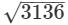= 56m

QUESTION: 3

### The circular grass lawn of radius 35 mhas a path of width 7 m around it on the outside. What is the area of the path?

Solution: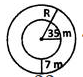r = 35 m; R = 35+7=42 m
Area of circular path
= π(R+r)(R−r)
= 22/7(42+35)(42−35) = 1694m2

QUESTION: 4

The difference between the circumference and radius of a circle is37 m. What is the circumference of the circle?

Solution:

2πr − r = 37

r = 37/2π−1 =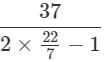= 7m

Circumference = 2πr = 2 × 22/7 × 7 = 44m

QUESTION: 5

How many plants (approximately) will be there in a circular bed whose outer edge measures 30 cm allowing 4 cm2 for each plant?

Solution:

Circumference = 30 cm Area

= C2/4π =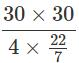=71.6cm2

Number of plants = A4 = 71.64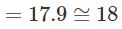QUESTION: 6

When the circumference and area of a circle are numerically equal, what is the diameter numerically equal to?

Solution:

Area of circle = Circumference of circle
πr= 2πr = 2 ⇒ d = 2 + 2 = 4units

QUESTION: 7

If the ratio of circumferences of two circles is 4:9, what is the ratio of their areas?

Solution:
QUESTION: 8

If the area of a circle is A, radius is r and circumference is C, which of the following is true?

Solution:
QUESTION: 9

What is the breadth of a rectangular sheet of perimeter 100 cm and length 35 cm?

Solution:
QUESTION: 10

A wall hanging is in the shape given in the figure. Find its perimeter.Solution:

The perimeter of the wall hanging is given by the sum of circumferences of the 4 semicircles −4 x diameter. Clearly, the diameter of each semicircle is 14 cm. The required perimeter

= 2 × circumference of circle of radius 7 cm.

= 2×2 × 22/7 × 7 = 88cm

QUESTION: 11

A wire bent in the form of a circle of radius 42 cm is again bent in the form of a square. What is the ratio of the regions enclosed by the circle and the square?

Solution:

Length of wire = 2π × 42 = 84πcm
Let x be the side of the square. We have,  4x = 84π ⇒ x = 21π
Area of the circle: Area of the square
= π(42)2:(21π)2
= 4:π = 4:22/7 = 14:11

QUESTION: 12

The length and the breadth of a rectangular piece of land are 400 m and 250 m respectively. What is the cost of the land at Rs. 1000 per square metre?

Solution:

∴ Area = 400 × 250m2
= 100000 m2
Cost of the land per square metre
= Rs.1000
∴  Cost of total land
100000 × Rs.1000 = Rs.10 crores

QUESTION: 13

In the given figure, ABCD is a parallelogram. DL⊥AB and DM⊥BC. If AB = 18cm,BC = 12cm and DM = 10cm,find DL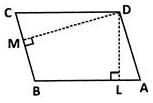Solution:

Here we equate the areas, i.e., AB×DL = BC×DM

⇒ 18×DL = 12×10
⇒ DL = (12×10)/18 = 20/3 =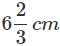QUESTION: 14

In the following figure, ABCD is a parallelogram. DL⊥AB and AB =13 cm = AD . If the area of parallelogram is 156 cm2, find AL.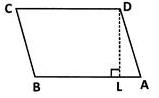Solution:

We have, area =156 cm2
⇒ b×h = 156
AB×DL=156
DL = 156/AB =156/13 = 12cm
∴ AL =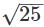=5cm

QUESTION: 15

The length and breadth of a rectangular hall in a model are 0.4 m and 30 cmrespectively. What is the distance between the opposite corners of the wall in the model?

Solution:

Distance between two opposite corners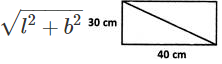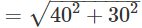= 50cm

QUESTION: 16

In the figure given, PR = 22 cm,SN = MQ = 3cm. What is the area of PQRS?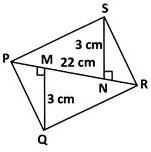Solution:
QUESTION: 17

Find the area of a verandah 2.25 m wide constructed outside a room 5.5 m long and 4 m wide.

Solution:
QUESTION: 18

The longer side of a parallelogram is 81 cmand the corresponding altitude is16 cm. If the length of shorter side is 24 cm,what is the altitude corresponding to the shorter side?

Solution:
QUESTION: 19

One side of a parallelogram is 2.4 dam and its area is 576 m2. Find the corresponding altitude.

Solution:

2.4 dam =2.4×10 = 24m
(Since 10 m = 1 dam.)
We have, area = 576 m2
⇒ 24 × altitude = 576
∴ altitude = 576/24 = 24m

QUESTION: 20

A field is in the form of a parallelogram whose base is 420 m and altitude is 3.6 dam. Find the cost of watering the field at 10 paise per sq m.

Solution: